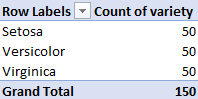# How to compute summary statistics (in Excel)

See all solutions.

The phrase “summary statistics” usually refers to a common set of simple computations that can be done about any dataset, including mean, median, variance, and some of the others shown below.

## Solution

Let’s assume you have some data in an Excel workbook. We show the first 10 rows the famous example dataset “iris” below, but we assume you are applying what we cover here to your own real data.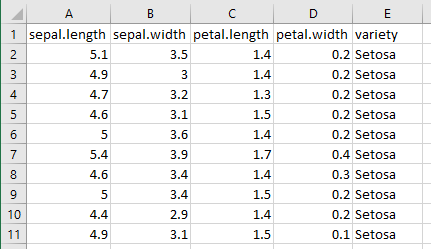To compute descriptive statistics, you will need the Data Analysis Toolpak. If you’ve never enabled it before, see these instructions from Microsoft on how to do so.

On the Data tab, click the Data Analysis button, shown below.From the list of tools it provides, choose Descriptive Statistics, as shown below, then click OK.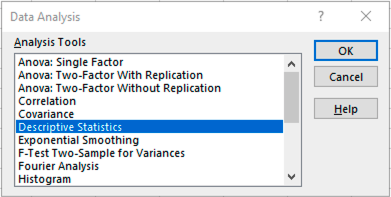Highlight as input only the numeric columns in your data, because the Data Analysis Toolpak cannot summarize other kinds of input. (Do not include column headers in your selection.) Then check the “Summary statistics” checkbox, as shown below. Then click OK.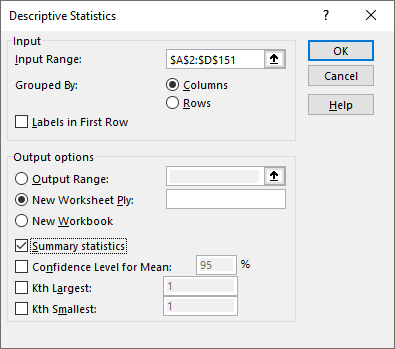Excel will create a new sheet that reports the mean, median, variance, and more, for each column, as shown below.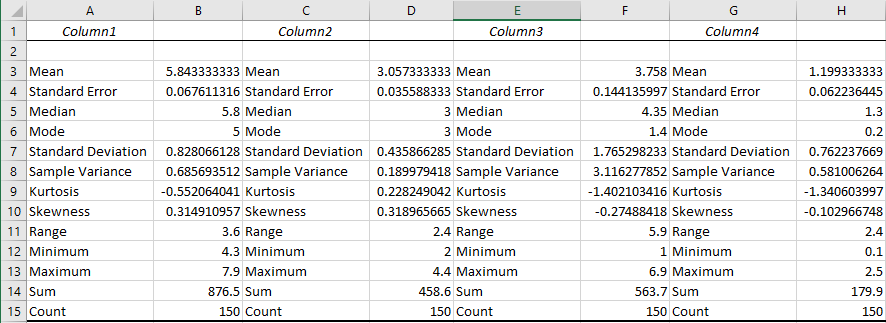To get a report of the unique values in your column and the frequency of each, you can use a pivot table. Place your cursor anywhere in your data, then on the Insert tab, choose Pivot Table, as shown below.Drag any column’s name from the list of pivot table fields down into both the Rows and Values areas, as shown below.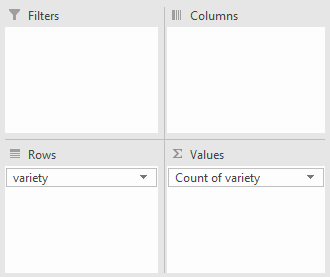If the column is numeric, then in the drop-down under Values, choose “Value field settings…” and change Sum to Count, then click OK. This is not necessary for non-numeric columns. (See how to summarize a column for an example.)

Your pivot table will now contain the desired report. In the iris dataset used in this example, there were precisely 50 observations of each variety.